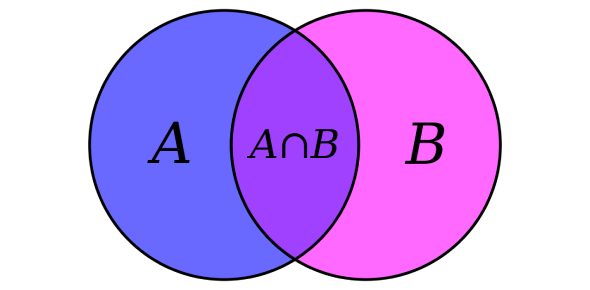# Set Quiz Questions And Answers

Approved & Edited by ProProfs Editorial Team
At ProProfs Quizzes, our dedicated in-house team of experts takes pride in their work. With a sharp eye for detail, they meticulously review each quiz. This ensures that every quiz, taken by over 100 million users, meets our standards of accuracy, clarity, and engagement.
| Written by Debyon
D
Debyon
Community Contributor
Quizzes Created: 1 | Total Attempts: 18,218
Questions: 10 | Attempts: 18,509SettingsCan you score high with these Set quiz questions and answers? This quiz will test how much you know about Sets. It will consist of various types of questions related to sets. Let us see how well you perform and how many of these questions you manage to answer correctly. Give your best, and enjoy playing this quiz. Best of luck to you with this amazing test! Let us go now!

• 1.

### A = { 1,5,3,7,9} and B = {2,3,4,5,6} then A n  B = ___

• A.

{ 1,5,3,7,9}

• B.

{2,3,4,5,6}

• C.

{ 3, 5}

• D.

None of these

C. { 3, 5}
Explanation
The intersection of sets A and B is the set of elements that are common to both sets. In this case, the common elements between A and B are 3 and 5. Therefore, the correct answer is { 3, 5}.

Rate this question:

• 2.

### A = {1,2,3,4,5,6} and B = {2,4,6} Which of the following represent A  U B?

• A.

{3,4,6}

• B.

{2,4,6}

• C.

{1,10,90}

• D.

{1,2,3,4,5,6}

D. {1,2,3,4,5,6}
Explanation
The union of sets A and B, denoted as A U B, represents the combination of all elements from both sets. In this case, set A contains the numbers 1, 2, 3, 4, 5, and 6, while set B contains the numbers 2, 4, and 6. Therefore, the union of A and B would be the set that includes all the numbers from both sets, which is {1, 2, 3, 4, 5, 6}.

Rate this question:

• 3.

### P u B = { 2,2, 4, 6, 8, 18,15,3, 47}

• A.

True

• B.

False

B. False
Explanation
The given statement is false because the set P u B contains multiple occurrences of the number 2, which violates the definition of a set. In a set, each element should be unique and not repeated. Therefore, the correct answer is false.

Rate this question:

• 4.

### The Venn Diagram shows a Disjoint set

• A.

True

• B.

False

A. True
Explanation
The Venn Diagram shows a disjoint set because there are two separate circles that do not overlap or share any elements. In a disjoint set, the elements in one set are completely separate from the elements in the other set.

Rate this question:

• 5.

### Every set must have numbers.

• A.

True

• B.

False

B. False
Explanation
This statement is incorrect. Every set does not have to have numbers. A set can contain any type of elements, including numbers, letters, or even objects. Therefore, the correct answer is False.

Rate this question:

• 6.

### A disjoint set is

• A.

Two sets that have no members in common

• B.

The set of all the members in both sets without creating any of the members in the sets

• C.

The set of all members that are common to both sets

• D.

The set of all the members that are partially common

A. Two sets that have no members in common
Explanation
A disjoint set refers to two sets that have no members in common. This means that there is no element that is present in both sets. In other words, the intersection of the two sets is an empty set.

Rate this question:

• 7.

### The __________ of sets is the set of all the members of both sets without repeating any of the members in the sets.

• A.

Union

• B.

Pair

• C.

Numbers

• D.

None of these

A. Union
Explanation
The union of sets is the set that contains all the elements from both sets without any repetition. It combines the elements of both sets into a single set, ensuring that no duplicate elements are included.

Rate this question:

• 8.

### The symbol U is used to indicate the intersection of sets.

• A.

True

• B.

False

B. False
Explanation
The symbol U is not used to indicate the intersection of sets. The correct symbol for the intersection of sets is ∩. The symbol U is used to indicate the union of sets. Therefore, the given statement is false.

Rate this question:

• 9.

### The intersection of two sets is the set of all members that are common to both sets.

• A.

True

• B.

False

A. True
Explanation
The statement is true because the intersection of two sets is defined as the set that contains all the elements that are present in both sets. In other words, it is the set of common elements between the two sets. If there are no common elements, then the intersection would be an empty set.

Rate this question:

• 10.

### A = {1,2,3} and B = {4,5,6} then A n B = ______?

• A.

{}

• B.

Prime numbers

• C.

Universal set

• D.

None of theseBack to top